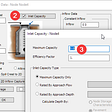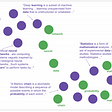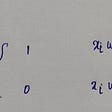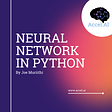# How Neural Network Works

A neural network is a computational structure that connects an input layer to an output layer. This computational structure is used in training deep learning models that can easily outperform any classical machine learning algorithm. As a data science beginner, you must have heard about neural networks before, but do you know how a neural network works? If you want to know, then this article is for you. In this article, I will explain how a neural network works.

# Here’s How a Neural Network Works

A Neural Network consists of three types of layers:

1. one input layer
2. one or more hidden layers
3. one output layer

Let’s understand how a neural network works with an example of Image Classification. To classify images using a neural network, we will first feed the neural network with the pixel values of images. The first layer of a neural network is the input layer that receives the data as input.

The second layer of a neural network is the hidden layer, responsible for all the calculations to learn from the features of the input data. There are only three layers in a neural network, but the number of hidden layers can be increased. The more complex your problem, the more hidden layers are preferred. Typically, a neural network with 1–2 hidden layers will work in most deep learning problems, but if the data has a lot of features to learn from, you can choose 3–5 hidden layers.

The last layer of a neural network is the output layer which classifies the data and provides the final output. The result given by an output layer is controlled by an activation function. The activation function is placed after the hidden layer and used to calculate the weighted sum of inputs and biases, used to determine whether a neuron should be activated or not.

So the first layer or the input layer of a neural network receives the input, the second layer or the hidden layers learn from the features of the input data, and the third layer or the output layer provides the output controlled by an activation function.

# Summary

So a neural network is a computational structure used to train deep learning models that can easily outperform any classical machine learning algorithm. A neural network consists of three types of layers:

1. one input layer that receives the data
2. one or more hidden layers that perform all the calculations to learn from the features
3. one output layer that provides the final output

I hope you liked this article on how a neural network works. Feel free to ask valuable questions in the comments section below.

## More from Aman Kharwal

I write stories behind the data📈 | https://www.instagram.com/the.clever.programmer/

Love podcasts or audiobooks? Learn on the go with our new app.

## Let’s See How a Computer Sees…## One Hundred Years of Solitude Meets Google Translate## How to model a sealed manhole that can overflow in XPSWMM?## TOP2VEC: New way of topic modelling## Decision Tree Classification in Python with Scikit-Learn## How to train with ArcFace loss to improve model classification accuracy## ARTIFICIAL NEURAL NETWORKS## Object Detection With OpenCV: Step by Step## Aman Kharwal

I write stories behind the data📈 | https://www.instagram.com/the.clever.programmer/

## Understanding Perceptron## Parametric and Nonparametric Machine Learning Algorithm## Machine Learning 101:Introduction## Neural Networks